# Catenoids

In cylindrical coordinates, let r(z), -a z a, denote the surface of a cylinder of varying radius. We wish to find the function that minimizes, at least locally, the area of this surface subject to the constraint that the radius at the two endpoints is fixed (at, say, ra). The surface area is given by: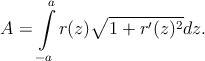Using calculus of variations we can discover the differential equation satisfied by the minimal surface. To this end, we look at the differential δA of area associated with a differential δr(z) of the radius function and set this differential to zero: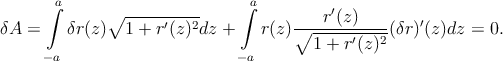Integrating the second integral by parts, we get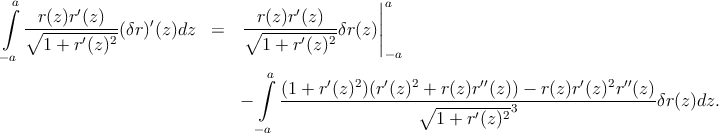Ignoring for the moment the end-conditions and combining the two integrals, we get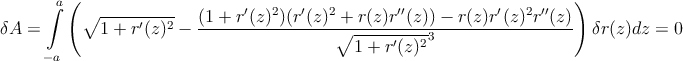This integral must vanish for all choices of differential function δr(z). Hence, the multiplying factor must vanish: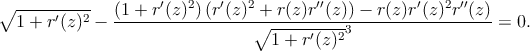It is easy to simplify this equation to read: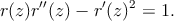It is also easy to check that the function r = cosh(z) satisfies this differential equation. Any cylinder corresponding to the cosh function is called a catenoid.

Of course, r = c cosh(z∕c) satisfies the equation too for any constant c. Thinking physically, this is obvious as it’s just a change of units, r r∕c, z z∕c, and clearly the shape of the minimal surface should not depend on units.

Since units are irrelevant, the interesting quantity is the ratio r∕z at the two endpoints (ra∕a). For the cosh function this ratio is always at least 1.50888—see Fig. 1.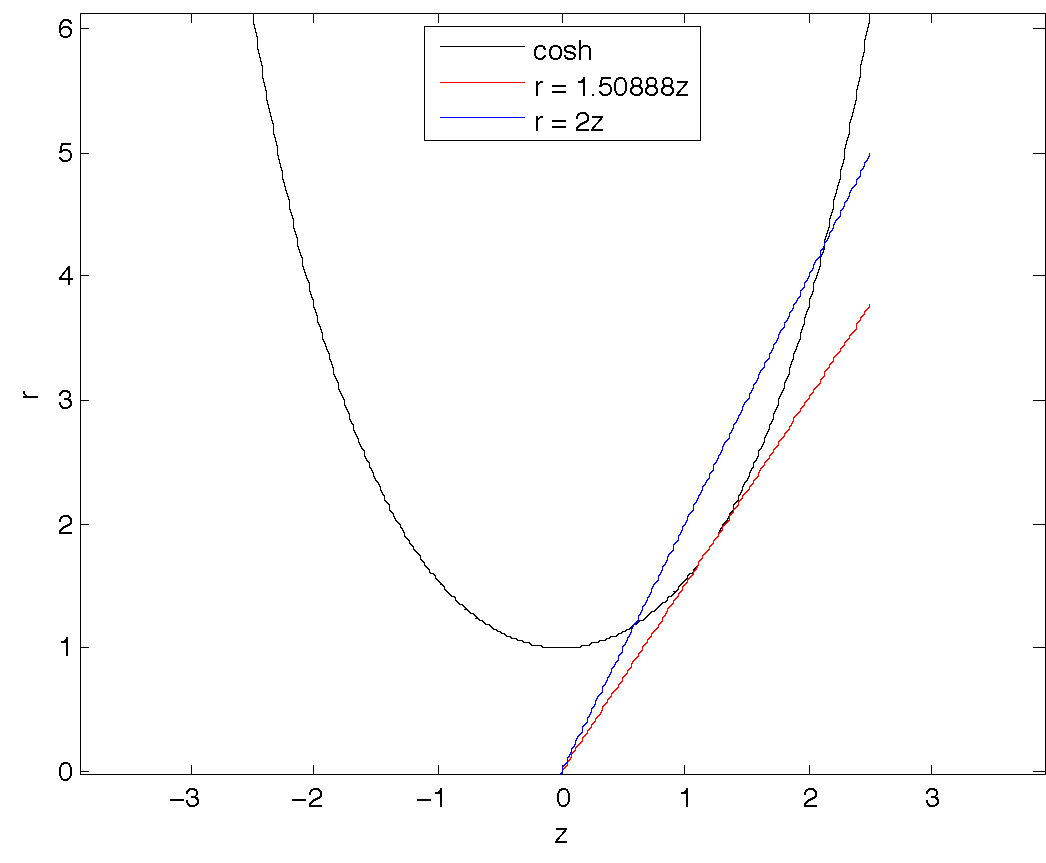Fig. 1.—: The function r = cosh(z).

For values of this ratio larger than this critical value, there are two (locally) minimal surfaces having that aspect ratio. See, e.g., the two intersections of the blue (r = 2z) line with the cosh function in Fig. 1.

For values of this ratio smaller than 1.50888, there is no cylindrical minimal surface having a cosh function defining its boundary. In fact, for such ratios, numerical studies suggest that the minimal surface is just two filled-in circles at the endpoints. In other words,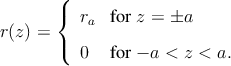In fact, for all aspect ratios smaller than 1.9758, this two-disk solution has smaller surface area than the corresponding catenoid. So, even when the catenoid is locally optimal, it can fail to be the global optimum.